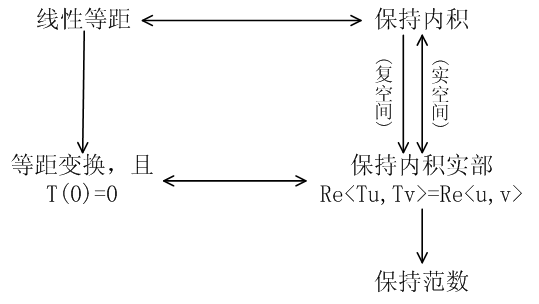# Linear Algebra Done Right第九章注记和部分习题

1 定理 9.9 的另一种证明

$$p(A_1),\dots,p(A_m)$$

3 设 $$A$$ 是分块对角矩阵 $A=\begin{pmatrix}A_1&& 0 \\ &\ddots& \\ 0&&A_m\end{pmatrix}$ 其中 $$A_j$$ 为方阵，证明 $$A$$ 的特征值的集合等于 $$A_1,\dots,A_m$$ 特征值集合的并集。
4 设 $$A$$ 是分块对角矩阵$A=\begin{pmatrix}A_1&&* \\ &\ddots& \\ 0&&A_m\end{pmatrix}$

8 证明不存在 $$T\in\mathcal L(\mathbf R^7)$$ 使得 $$T^2+T+I$$ 是幂零变换。

（10题可利用8题的结论，11题利用10题的结论）

14 设 $$V$$ 是 2 维向量空间而 $$T\in\mathcal L(V)$$，证明如果
$$\begin{pmatrix}a&b \\ c&d\end{pmatrix}$$

|

# 【转】丘成桐：漫谈微分几何

，好象是不太容易培养出这种气氛来。假如你旁边的朋友或同学跟你谈的都是其它的问题，譬如说股票涨了或跌了或其它问题，久而久之，你大概对于做学问也没有很大的兴趣，所以培养做学问的态度与你交的朋友、跟的老师的关系很大。如果你们时常讨论学术上的问题，你就不会觉得自己很孤单，能够激励你对数学上有更大的兴趣。假如你自暴自弃，就是说你认为自己不能够在数学上做研究，不能够在数学上达到贡献的话，你永远也达不到，而且同时也影响到你旁边的朋友，使得大家都不能向前走。我们晓得许多出名的数学家甚至在牢里也可以写一些出名的文章，倒不是你永远关在牢里就能做好的文章，是说人在最困难的时候也可以做研究。除了气氛很重要外，你也需要得到先进的支持，从前我们念中学的时候，念了很多关于做学问的方法，从前觉得很好笑，以后念书念得多了以后就觉得这些很重要，事实上这些是很重要的经验。有句话说「学而不思则罔，思而不学则怠」，你单是学而不想是不行的，你单是想而不学也是不行的，这两句话看起来很简单，其实就是怎么分配你的学习跟思想，这是一个很微妙很重要的问题。一个人无论你多用功多天才，你假如不将前人做过的东西去体验去学习，是不可能做好的。这道理很简单，一个人的智能有限，我们不可能与前面十年、五年所有人做过的加起来的智能相比，我们要靠前人的经验，要靠他们的启发，才能够向前迈进，虽然有人自夸的讲比他们加起来都行，我不相信这种情形，也没见过这种情形。所以出名的贡献如爱因斯坦、牛顿的贡献，也是在前人的成果方面再向前走一大步或一小步。所以学是一定要的，可是如果你学过这个东西以后而不去思考，不去消化，就算你可以考第一，考一百分，但是你不想是绝对没有用的。我们看过很多出名的天才，十二岁就拿到学士学位，甚至拿了很高分，可是往往我们看不出他以后的成就。为什么很多所谓的天才在以后的科学发展里没有任何的贡献？这是因为他们没有思考，没有思考在科学上完全不会引起任何的波澜、任何的贡献，对于整个科学完全没有好处。所以学了以后一定要思考，怎么分配你的学习跟思考就往往要有导师的帮忙或是同学的帮忙。所谓的帮忙并不是说老师跟你讲你应当这么做或应当怎么做，这样往往是没有很大的效果，所以我刚刚讲的气氛很重要。从人家用功的程度或是讲话的态度的启发，或是讲话的时候能够去听，追根出什么东西来，从它而得到很大的帮助。从前我到柏克莱去念研究所时，我花了很多功夫去听很多不同的科目，有些人觉得很奇怪，为什么我会去听那些课？我觉得这些课对我有好处，过了几十年后我还是觉得有好处。有些课在我去听的当时可能不懂，可是听了还是觉得有好处，因为一个人的脑袋的想法并不是那么简单的，有时候某些东西当时可能不懂，可是慢慢的就能领悟很多东西。我举例来讲，我做博士论文的时候，我刚好要用到群论的东西，当时我问过许多专家，但是都不懂，我突然想到从前在某一课上听过一个有关这方面的论文，我忘了当时讲什么课，但我记得大概在那里可以找这方面的文章，所以我花了2天的时间在图书馆，结果给我找到差不多是我所要的文章。假如当初不去听这门课的话，我完全没有这个机会，所以有时候听一门不懂的课，有很多不同的帮助，所以很多研究生我跟他们讲，你们去听课不一定要懂，你坐在那边总比不坐在那边好，你不坐在那边的话，你完全不可能知道有其它的方法。

# Linear Algebra Done Right第八章注记和部分习题

1 $$\dim\mathrm{null}\,T^k$$ 随着 k 的增加量

$$\dim\mathrm{null}\,T^k$$ 是 k 的单调递增函数，而且当 k 达到某一个数值之后，$$\dim\mathrm{null}\,T^k$$ 就恒定不变了。但是书上并没有说它的增加有何规律，是每次都增加相同的维数，还是增加的维数可能有变化？换句话说，$$\dim\mathrm{null}\,T^{k+1}-\dim\mathrm{null}\,T^k$$ 有什么规律？它是恒定不变的，还是忽大忽小的，或是有什么别的规律没有？

3 引理 8.40 的另一种证明

a) $$(v_1,Nv_1,\dots,N^{m(v_1)}v1,\dots,v_k,Nv_k,\dots,N^{m(v_k)}v_k)$$ 是 $$V$$ 的基底；
b) $$(N^{m(v_1)}v1,\dots,N^{m(v_k)}v_k)$$ 是 $$\mathrm{null}\,N$$ 的基底。

3 设 $$T\in\mathcal L(V)$$，m 是正整数，且 $$v\in V$$ 是满足 $$T^{m-1}v\not=0$$ 但 $$T^mv=0$$ 的向量。证明 $$(v, Tv, T^2v,\dots,T^{m-1}v)$$ 线性无关。

5 设 $$S,T\in\mathcal L(V)$$。证明如果 $$ST$$ 是幂零的，那么 $$TS$$ 也是幂零的。

13 设 $$V$$ 是 n 维复向量空间，且 $$T\in\mathcal L(V)$$ 满足 $$\mathrm{null}\,T^{n-2}\not=\mathrm{null}\,T^{n-1}$$。证明 $$T$$ 至多有两个不同的特征值。

20 设 $$T\in\mathcal L(V)$$ 是可逆变换。证明存在多项式 $$p\in\mathcal P(F)$$ 使得 $$T^{-1}=p(T)$$。

28 设 $$a_0,\dots,a_{n-1}\in\mathbf C$$，某线性变换在标准基底下的矩阵是 $\begin{pmatrix}0&&&&&-a_0 \\ 1&0&&&&-a_1 \\ &1&\ddots&&&-a_2 \\ &&\ddots&&&\vdots \\ &&&&0&-a_{n-2} \\ &&&&1&-a_{n-1}\end{pmatrix}$ 找到它的最小多项式与特征多项式。

30 设 V 是复向量空间，且 $$T\in\mathcal L(V)$$。证明 $$V$$ 不能分解为 $$T$$ 的非平凡不变子空间的直和当且仅当 $$T$$ 的最小多项式形如 $$(T-\lambda I)^{\dim V}$$，其中 $$\lambda\in\mathbf C$$。

|

# Linear Algebra Done Right第七章注记和部分习题

1 命题 7.2 为何在实数空间不成立？

2 $$U$$ 是线性算子 $$T$$ 的不变子空间，那么 $$T^*|_U=(T|_U)^*$$ 是否成立？

$$T=\begin{pmatrix}1&1\\ 0&1\end{pmatrix}$$

$$\forall v, \langle Tv,w\rangle=\langle v,T^*w\rangle$$

$$\forall u\in U, \langle Tu,w\rangle=\langle u,(T|_U)^*w\rangle$$

3 实空间变换矩阵的分块上三角化与实空间的正规、自伴算子谱定理

4 自伴算子乘积什么时候是自伴的？

$$S=\begin{pmatrix}0&1\\ 1&0\end{pmatrix},T=\begin{pmatrix}1&0\\ 0&2\end{pmatrix}$$

$$ST=\begin{pmatrix}0&2\\ 1&0\end{pmatrix}\not=\begin{pmatrix}0&1\\ 2&0\end{pmatrix}=TS$$

$$V=\mathrm{null}\,(T-\lambda_1I)\oplus\mathrm{null}\,(T-\lambda_2I)\oplus\dots\oplus\mathrm{null}\,(T-\lambda_mI)$$

$$\displaystyle TSv=\sum_{i=1}^mTSv_i=\sum_{i=1}^mSTv_i=STv$$

5 正定算子的平方根

$$\begin{pmatrix}\cos\theta&\sin\theta\\ \sin\theta&-\cos\theta\end{pmatrix}$$

$$I$$ 的上述形式的平方根都是自伴的，$$I$$ 也有非自伴的平方根，比如，只要 $$a^2+bc=1$$，那么
$$\begin{pmatrix}a&b\\ c&-a\end{pmatrix}^2=I$$

$$\begin{pmatrix}\lambda_1&0\\ 0&\lambda_2\end{pmatrix}$$

$$\begin{pmatrix}a&b\\ c&d\end{pmatrix}^2=\begin{pmatrix}a^2+bc&b(a+d)\\ c(a+d)&d^2+bc\end{pmatrix}=\begin{pmatrix}\lambda_1&0\\ 0&\lambda_2\end{pmatrix}$$

6 等距变换几个相似概念之间的关系

1) $$T$$ 是等距变换，即 $$\forall u,v\in V,\|Tu-Tv\|=\|u-v\|$$
2) $$T$$ 保持范数，即 $$\forall u\in V,\|Tu\|=\|u\|$$
3) $$T$$ 保持内积，即 $$\forall u,v\in V,\langle Tu,Tv\rangle=\langle u,v\rangle$$$$\|Tku-kTu\|^2=\langle Tku,Tku\rangle-\bar k\langle Tku,Tu\rangle$$$$-k\langle Tu,Tku\rangle+k\bar k\langle Tu,Tu\rangle=0$$

$$\|Tu-Tv\|^2=\|Tu\|^2-2\mathrm{Re}\,\langle Tu,Tv\rangle+\|Tv\|^2$$
$$\|u-v\|^2=\|u\|^2-2\mathrm{Re}\,\langle u,v\rangle+\|v\|^2$$

 侯维民. 关于正交变换两种定义方式的探讨 [J]. 高等数学研究, 2005, 8(1): 44-45.
 [美] Peter.D.Lax. 线性代数及其应用 [M]. 傅莺莺，沈复兴，译. 北京：人民邮电出版社，2009.

7 极分解的另一种形式

5 证明如果 $$\dim V\ge 2$$，那么所有 $$V$$ 上的正规算子的集合不是 $$\mathcal L(V)$$ 的子空间。

$$\begin{pmatrix}2&1\\ -1&2\end{pmatrix}+\begin{pmatrix}2&1\\ 1&2\end{pmatrix}=\begin{pmatrix}4&2\\ 0&4\end{pmatrix}$$

7 证明如果 $$T\in\mathcal L(V)$$ 是正规算子，那么对所有正整数 k，有 $$\mathrm{null}\,T^k=\mathrm{null}\,T, \mathrm{range}\,T^k=\mathrm{range}\,T$$。

14 设 $$T\in\mathcal L(V)$$ 是自伴算子，$$\lambda\in\mathbf F, \epsilon>0$$，证明如果存在向量 $$v\in V$$ 满足 $$\|v\|=1$$ 且 $$\|Tv-\lambda v\|<\epsilon$$，那么 $$T$$ 有特征值 $$\lambda’$$ 满足 $$|\lambda-\lambda’|<\epsilon$$。

$$\min_i |\lambda_i-\lambda|^2\le\sum_{i=1}^n|\lambda_i-\lambda|^2v_i^2=\|Tv-\lambda v\|^2<\epsilon^2$$

18 证明如果 $$T$$ 是 $$V$$ 上的半正定算子，那么对任意正整数 k，$$T^k$$ 也是半正定的。

$$\langle T^kv,v\rangle=\langle T^{\frac{k}{2}}v,T^{\frac{k}{2}}v\rangle\ge 0$$

$$\langle T^kv,v\rangle=\langle TT^{\frac{k-1}{2}}v,T^{\frac{k-1}{2}}v\rangle\ge 0$$

24 设 $$T\in\mathcal L(V)$$，$$S\in\mathcal L(V)$$ 是 V 上的线性等距变换， $$R\in\mathcal L(V)$$ 是 V 上的半正定算子且 $$T=SR$$，证明 $$R=\sqrt{T^*T}$$。

27 证明或给出反例：如果 $$T\in\mathcal L(V)$$，那么 $$T^2$$ 的奇异值是 $$T$$ 的奇异值的平方。

|

# 理解复数域上的向量空间（第一篇）

\begin{aligned}\langle Tu,w\rangle=&\frac{\langle T(u+w),u+w\rangle-\langle T(u-w),u-w\rangle}{4}\\ &+\frac{\langle T(u+iw),u+iw\rangle+\langle T(u-iw),u-iw\rangle}{4}i\end{aligned}